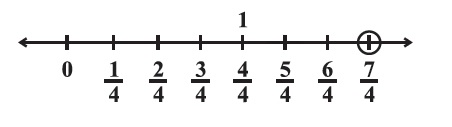# vs.eyeandcontacts.com

## Chapter 1 Rational Numbers Exercise 1.2

Question 1: Represent these numbers on the number line.
(i) 7/4(ii) -5/6Question 2: Represent -2/11, -5/11, -9/11 on a number line.Question 3: Write five rational numbers which are smaller than 2.

2 = 2/1 × 10/10 = 20/10
= 0/10, 5/10, 7/10, 11/10, 15/10

Question 4: Find the rational numbers between -2/5 and 1/2.

-2/5 and 1/2
LCM of 2, 5 = 10
-2/5 × 2/2 = -4/10
1/2 × 5/5 = 5/10
= -4/10 × 10/10 = -40/100
= 5/10 × 10/10 = 50/100
= -40/100, -39/100, -38/100, -37/100, -36/100, -35/100, -34/100, 0/100, 10/100, 20/100, 30/100, 50/100

Question 5: Find five rational numbers between.
(i) 2/3 and 4/5

2/3 and 4/5
LCM of 3, 5 = 15
2/3 × 5/5 = 10/15
4/5 × 3/3 = 12/15
= 10/15 × 10/10 = 100/150
= 12/15 × 10/10 = 120/150
= 101/150, 102/150, 103/150, 104/150, 105/150

(ii) -3/2 and 5/3

-3/2 and 5/3
LCM of 2, 3 = 6
-3/2 × 3/3 = -9/6
5/3 × 2/2 = 10/6
= -9/6 × 10/10 = -90/60
= 10/6 × 10/10 = 100/60
= -89/60, -88/60, -87/100, -86/100, -85/100

(iii) 1/4 and 1/2

1/4 and 1/2
LCM of 2, 4 = 8
1/4 × 2/2 = 2/8
1/2 × 4/4 = 4/8
= 2/8 × 10/10 = 20/80
= 4/8 × 10/10 = 40/80
= 39/100, 38/100, 37/100, 36/100, 35/100

Question 6: Write five rational numbers greater than -2.

-2/1 × 10/10 = -20/10
= -19/10, -18/10, -17/10, -16/10, -15/10

Question 7: Find ten rational numbers between 3/5 and 3/4.